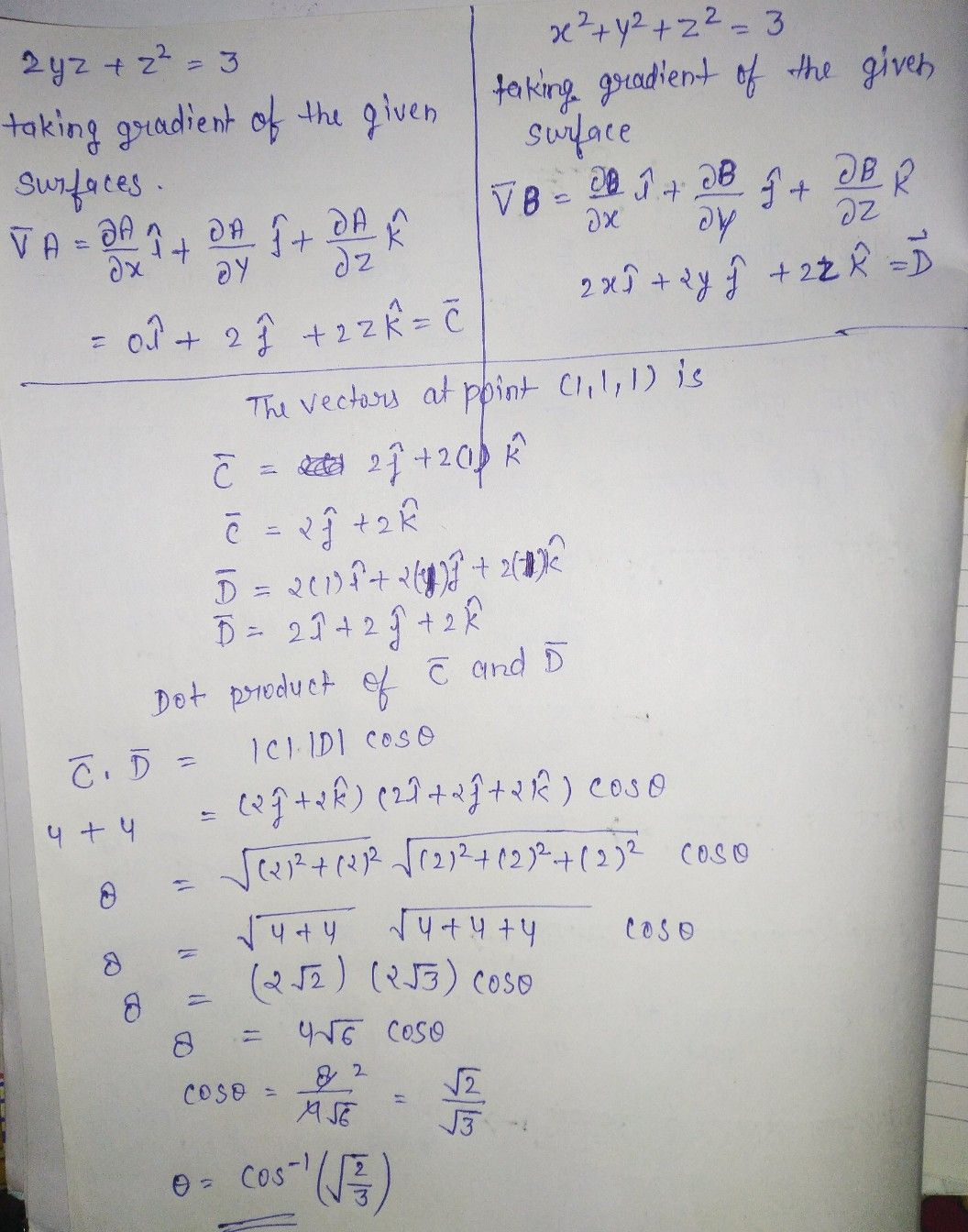Symbol
ProblemDetermine the angle between the surfaces $2yz+z^{2}=3$ and $x^{2}+y^{2}+z^{2}=3$ at the point(1, $\left(1,$ $1,$ $1\right)$
Calculus
Search count: 108
SolutionQanda teacher - AnkitaIf you have any doubts please do let me know dear. if you are satisfied with my answer please do LIKE and give a 5 STAR RATING and coins as THANK U GIFT..! ALL THE BEST..!!!?Student
tysm maamQanda teacher - Ankita
plz rate me 5 star ?Student
sure